# GRAPH MODELS

The necessity to use a graph model to represent multidimensional aggregate data was a natural consequence of the fact that the use of 2-D table representation had several deficiencies. 2-D table representation existed, and still continues to be practiced by statisticians today, because statistical data have been historically presented on paper. Using a 2-D representation requires squeezing the multidimensional space into two dimensions. This is typically done by arbitrarily choosing several of the dimensions to be represented as rows and several as columns. In general, 2-D representation of multidimensional data forces a (possibly arbitrary) choice of two hierarchies for the rows and columns (Shoshani, 1997). The apparent conclusion is that a proper graphical model should retain the concept of multi-dimensionality and represent it explicitly, as mentioned in Rafanelli & Shoshani (1990), where the authors referred this concept to the aggregate statistical data and proposed the STORM graph model.

2-D table representation changes some important semantic concepts. For example, classification hierarchies are represented in the same manner as multidimensional categories, while actually the classification hierarchy represents a single dimension. Instead, there is a fundamental difference between category attribute structure and multidimensionality. A category attribute represents a dimension of the aggregate data structure or an element (level) of the classification hierarchy which forms the dimension mentioned above. Usually, only the low-level elements of the classification relationship participate in the multidimensional space. Moreover, by necessity, more than one dimension must be represented by rows and columns if more than two dimensions exist in the dataset. This is accomplished by selecting an arbitrary order of the dimensions for rows and columns, as mentioned above. Again, the label of each multidimensional aggregate data structure (i.e., the title of the 2-D table) represents the summary measure for this dataset and can express other dimensions implicitly. For example, "Production of vegetables in California in 1995" says that this dataset has two additional dimensions: "state" and "year," where the instance values selected are the singletons "California" and "1995." In reality, the dimension names should really be "State of vegetable production" and "Year of vegetable production," because the phenomenon studied is "vegetable production." In this case, as we will see in the following, a primitive domain of the dimension (and of the different descriptive attributes in a classification hierarchy of a dimension) is defined as a common reference of the primary concept (in this case, "state" and "year"). Indeed, dataset may be only one "page" of a collection of pages, each representing another state and/or another year. There is also a mixing between categories and category instances. Depending on their use by the user, the same name can be used in both cases. For example, consider the classification hierarchy "professional category"-"profession" of the table "Employment in California" shown in Figure 1, and suppose that the instance domains have, respectively, <engineer, secretary, teacher> for professional category, and <chemical, civil> for "engineer" profession, <junior, executive> for "secretary" profession, and <elementary, intermediate> for "teacher" profession. In such cases, the category attributes and their values do not fit on a page or a screen, so that the representation spreads into multiple pages or screens. This confuses the global understanding of the multidimensional aggregate data. The problem is that the metadata on the multidimensional aggregate data has no separate representation. Furthermore, there should be a separation between structural metadata (the metaschema) and instance values (the meta-database).

Different graph models have been proposed in the literature: SUBJECT, described in Chan & Shoshani (1981); GRASS, described in Rafanelli & Ricci (1983); SAM*, described in Su (1983), in which there are seven types of associations, each with its own properties (i.e., structure, operations, and constraints), a G-relation and an algebra are formally defined; STORM, described in Rafanelli & Shoshani (1990); and ADAMO, described in Bezenchek, Rafanelli, & Tininini (1996a) and in Tininini, Bezenchek, & Rafanelli (1996). The distinctive feature of ADAMO in comparison to the other models for aggregate data is in general a major adherence to the aggregation process, and particularly the capability of:

• representing and distinguishing the implicit and explicit category attributes as well as the complex MAD;

• expressing, by simple and intuitive graphical notations, the summarizability characteristics of a category attribute and the marginal values of a MAD;

• expressing the hierarchies in the dimensions of a MAD along with the corresponding summarizability characteristics and marginal values;

• expressing the identification dependencies in the hierarchies of a MAD;

• representing the composite MAD in a compact form by introducing the alias nodes;

• handling the problem of integration among data supplied by different information sources.

The model utilizes two representation spaces, called, respectively, intentional and extensional space. Orthogonally to these spaces, two distinct representation levels are defined, called, respectively, A (for Aggregate data) and B (for Base concept) level, as shown in Figure 8.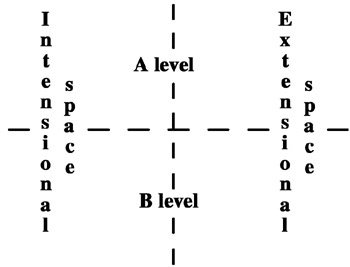Figure 8: Spaces and Levels of ADAMO

The intentional side of the A level allows a conceptual description of the MAD by means of tree-structures, whereas in the extensional side both the category attribute instances and the summary values are listed. The B level contains primitive concepts, namely primitive circle nodes, primitive phenomena, and primitive instances to which all the circle nodes, square nodes, and instances of the A level are linked. The B level allows two concepts sharing the same name as the A level to be distinguished when they are linked to distinct primitive concepts. Also, two concepts with different names at the A level (synonyms) are unified when they are both linked to the same primitive concept. In the A level a conceptual description of the MAD is given. The main advantage of the graphical approach is that it allows "at a glance" understanding of even very semantically complex multidimensional aggregate data. The conceptual description of a MAD is achieved by means of a tree-structure with variously shaped nodes, each of them in strict correspondence with one of the following concepts:

1. a simple circle node (O) represents a classification set (i.e., an explicit category attribute);

2. a slashed circle node (Ø) represents a union set (i.e., an implicit category attribute);

3. a triangle node (Δ) represents a cross-intersection among its child-nodes (note that the associativity of the operator Ä allows a triangle node to have more than two child-nodes);

4. a butterfly node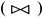represents the union set among its child-nodes;

5. a square node () represents the mapping f between the aggregation relations and the corresponding summary values, and also several other properties regarding the MAD such as the described fact, the aggregate function type, the summary type, the information source, the measure unit, and the counting unit.

For example, let us reconsider the table of Figure 2. The corresponding graphical representation by the ADAMO model is shown in Figure 9.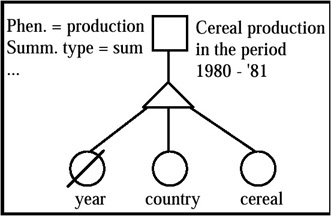ADAMO provides four graphical notations, shown in Figure 10, to express the summarizability characteristics of each MAD, namely to express that the category attribute A is (i) T-summarizable (totally summarizable); (ii) I-summarizable (Implicitly summarizable); (iii) non-summarizable due to overlaps among its base sets; and (iv) non-summarizable because the operation is meaningless.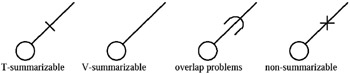Figure 10: Graphical Notations of Different Summarizability Characteristics

A MAD with marginal values can always be represented by a complex tree-structure with (at least) one union node. However, a concise notation has been introduced that allows the user to represent the marginal values by means of the structure corresponding to the non-marginal summary values. Let us consider for example the table outlined in Figure 11.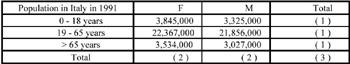Figure 11: Table Example

This MAD is conceptually represented by the ADAMO tree-structure in Figure 12. Each set of marginal values conceptually obtainable by summarizing a single category attribute is represented by a little black circle on the edge above the correspondent circle node.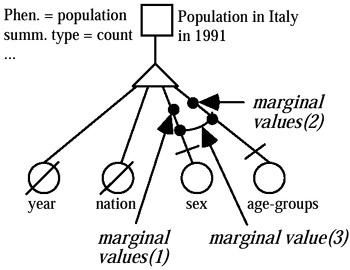Figure 12: Graphical Representation of Marginals and Implicit Dimensions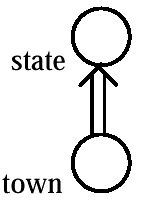Figure 13: Graphical Representation of the ID-Dependency

The set of marginal values obtainable by summarizing two or more category attributes is represented by an equal number of little black circles connected by an edge.

It often happens that the category attribute instances in a MAD are not uniquely defined due to homonymy. For example, in an aggregation hierarchy "state town," if the domain of state consists of the values New York, Wyoming, Texas, California, Arizona, Nevada, Oregon, and the domain of town includes the value Buffalo, this value is not uniquely defined because a city with such a name exists both in New York and in Wyoming. On the other hand, the pair of instances (state, town) is sufficient to univocally identify each instance of town, since it is known that no two towns with the same name exist in the same state. We say that between the category attributes town and state, an identification dependency exists. This is a very important piece of information, since it can greatly simplify the process of linking between the instances of the A level and the corresponding primitive instances of the B level. Figure 3 shows the ADAMO graphical representation of an identification (ID-) dependency (see Rafanelli & Shoshani, 1990).

We have already considered the use of butterfly nodes to define complex MADs. The introduction of a butterfly node is also required when a single MAD collects data obtained by two or more different aggregation processes, that is when it represents a composite MAD. The corresponding aggregation functions and/or facts are obviously distinct, and each needs to be represented by a distinct square node. Several square nodes are linked to the same upper butterfly node, opportunely labeled.

Let us consider for example the table of Figure 14, where both the population (classified by state and sex) and the average income (classified by state) are displayed.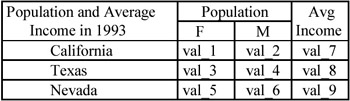Figure 14: Composite Table

The ADAMO representation is shown in Figure 15.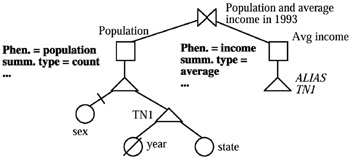Figure 15: The Graphical Representation of an Alias Node

Note that in this case the node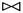represents the union of the underlying structures (MADs), rather than the union of aggregation sets. Another important observation refers to the alias node: an alias node is a shorthand and represents a reference to the entire structure, having as its root the original node, or simply to the entire domain of the original node, if it is a circle node.

Alias references are allowed only within the same composite MAD. Butterfly nodes, aliases, and marginals allow a very compact representation of the MAD (in Figure 16) to be obtained. This table fragment is taken from Bezenchek, Rafanelli, & Tininini (1996a) and represents population data in some U.S. towns. In this fragment the towns are grouped by state and two subtotals are also displayed, one representing the aggregate population of the listed towns, and the other representing the whole population of the state. The corresponding ADAMO tree-structure is that of Figure 17. The circle node town refers to the first term in brackets; the marginal symbol refers to the second term (as it represents the summarization of town, that is its implicitation with the explicitation of state), and the circle node ALIAS state refers to the last term.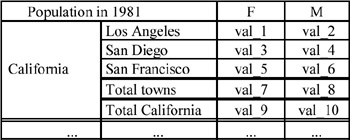Figure 16: Different Total Levels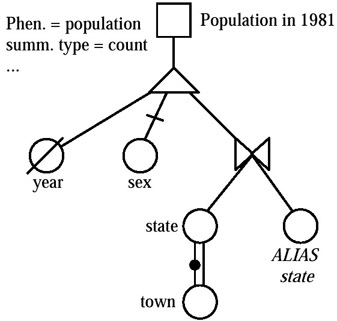Figure 17: Graphical Representation of Butterfly Node with Alias and Marginals

As stressed above, state is a virtual node needed to express both the fact that the towns are grouped by state, and also that the marginal values corresponding to the summarization of town are directly available in exact form.

Notice that, since state is needed both in virtual and in explicit mode (to express the entire state values), the ALIAS mechanism allows there to be two state circle nodes (one in the hierarchy and the other in leaf-position), again to define the instances of the corresponding attribute only once.

### Tabular Models

Tabular models are used both in SDB and OLAP. A 2D statistical table (as in Figure 4), with the adding of columns and rows that show summarization over rows and columns respectively, is an example of these models. These totals are often referred to by statisticians as "marginals" (because they appear on the "margins" of tables). The marginals are usually not included in the database if they can be derived. However, in situations where summarizability does not hold, it is necessary to store these values in the database as well. It is generally not efficient to compute the marginals for very large datasets. This is one of the most important problems addressed by OLAP research and it will be discussed in Chapter 9.

Another tabular representation stems from the popularity of the relational model. The advantage of this representation is its familiarity and the fact that it can be readily implemented on a relational system, as well as the possibility of integrating it with other data in the same model.

However, there are several problems with this representation:

1. There are no semantics associated with a relational table to distinguish between category and summary attributes.

2. There is no distinction between the attributes associated with the classification hierarchy and the dimensions.

3. The repetition of values in the columns relative to the descriptive attributes make it difficult to see the categories of each of these category attributes.

Furthermore, if the relation is implemented in a straightforward way, this layout is very wasteful of space since it stores the entire cross product. Consequently, summarization and query processing is slowed down.

Another issue for using this model is how to represent marginals. In Gray et al. (1996), the authors suggest using the reserved keyword value "ALL" to achieve this purpose. However, one would have to know which of the columns represent the dimension and which the summary attribute, since "ALL" cannot be applied to summary attributes. In addition, this solution does not deal with classification structures which exist in other relations if normalization is applied. With this construct, the authors have defined a "data cube" operator, which we will discuss in Chapter 5.

A relational representation that partially overcomes the above problems is used by an OLAP company (see MicroStrategy). An example of this representation is shown in Figure 20 for a hospital and patient procedures database (the figure is taken from Shoshani, 1997). They call it the "star model," since it has one relation that represents multidimensional space in the center and the other relations that represent dimensions around it.

As can be seen from Figure 18, this is an attempt to introduce the semantics of a MAD in the context of the relational model. By labeling relations as the "fact table" and "dimension tables," they make a distinction between summary data and the data associated with the dimensions. By placing the "fact table" visually at the center of the "star," they convey the multidimensional nature of this database. Furthermore, each dimension table can hold the category attributes of the classification structure for that dimension. For example, the "hospital" dimension table has attributes "city" and "state" that make up the classification structure. Many of the difficulties described previously still exist. Specifically:

1. The "fact table" still requires the storage of the entire cross-product.

2. It is not possible to know whether an attribute in the dimension tables is a "category attribute" (such as "type" in the "procedure" table) or a regular descriptive attribute (such as "name" in the "procedure" table).

3. It is not possible to structurally assess the hierarchical relationship of the attributes that make up the classification structure. For example, there is no structural information on whether "procedures" group into "types" and "type" into "branches," or whether "procedures" group into "types" and also "branches" independently of "types."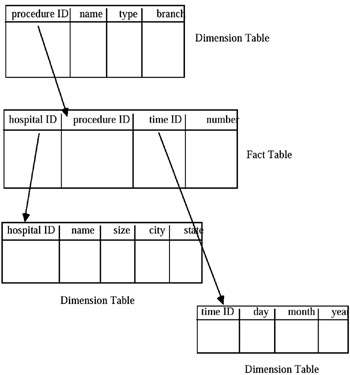Figure 18: Example of Star Model Representation

The data cube model is the most recent graphical representation of multidimensional data, and it is used in an OLAP environment. This representation is intended to be used only to convey concepts, but it cannot be used for real databases, for which one needs to resort to other representations such as the graphical or tabular representation described above. While the multidimensional space is clearly represented in this data cube model, the structure of the dimensions is not well presented. In spite of the above deficiencies, the data cube concept is useful in visualizing certain operations, such as "slice" and "dice."

In conclusion, we give a set of definitions regarding the OLAP terminology taken from The OLAP Council (1995):

Aggregate (or Consolidate): Multidimensional databases generally have hierarchies or formula-based relationships of data within each dimension. Consolidation involves computing all of these data relationships for one or more dimensions. Such relationships are normally summations, but any type of computation relationship or formula might be defined.

Multidimensional Array (cube): This is a group of data cells arranged by the dimensions of the data. An example of a two-dimensional array is a spreadsheet, with the data cells arranged in rows and columns, each being a dimension. An example of a three-dimensional array is a cube, a physical metaphor of a multidimensional visualization of the data. Each dimension of the cube forms a side. A higher dimensional array has no physical metaphor, but they organize the data in the same way that users think of their enterprise.

Calculated Member: This is a member of a dimension whose value is determined by other members' values (e.g., by the application of a mathematical or logical operation.). It is any member that is not an input member.

Cell: It is a single datapoint that occurs at the intersection defined by selecting one member from each dimension in a multidimensional array.

Children: They are the members of a dimension that are included in a calculation to produce a consolidated total for a parent member. Children may themselves be consolidated levels, which require that they have children. A member may be a child for more than one parent, and a child's multiple parents may not necessarily be at the same hierarchical level, thereby allowing complex, multiple hierarchical aggregations within any dimension.

Derived Data: Derived data is produced by applying calculations to input data at the time the request for that data is made, i.e., the data has not been pre-computed and stored on the database. The purpose of using them is to save storage space and calculation time, particularly for calculated data that may be infrequently called for or that is susceptible to a high degree of interactive personalization by the user.

Detail Member: A detail member of a dimension is the lowest level number in its hierarchy.

Dimension: This is a structural attribute of a cube that is a list of members, all of which are of a similar type in the user's perception of the data. A dimension acts as an index for identifying values within a multidimensional array. If one member of the dimension is selected, the remaining dimensions in which a range of members (or all members) are selected define a sub-cube. If all but two dimensions have a single member selected, the remaining two dimensions define a spreadsheet (or a "slice" or a "page"). If all dimensions have a single member selected, then a single cell is defined.

Drill-Down/Up: Drilling-down or up is a specific analytical technique whereby the user navigates among levels of data ranging from the most summarized (up) to the most detailed (down). The drilling paths may be defined by the hierarchies within dimensions or other relationships that may be dynamic within or between dimensions.

Formula: A formula is a database object, which is a calculation, rule, or other expression for manipulating the data within a multidimensional database Formulae define relationships among members. They are used by OLAP database builders to provide great richness of content to the server database. Formulae are used by end-users to model enterprise relationships. They are also used to personalize the data for greater visualization and insight.

Hierarchical Generation: Two members of a hierarchy have the same generation if they have the same number of ancestors leading to the top. Notice that the term generation and level are both necessary to describe sub-groups of dimension members, since, for example, although two siblings share the same parent and are, therefore, of the same generation, they won't be from the same level if one of the siblings has a child and the other doesn't.

Hierarchical Level: Members of a dimension with hierarchies are at the same level if, within their hierarchy, they have the same maximum number of descendants in any single path below.

Hierarchical Relationships: Any dimension's members may be organized based on parent-child relationships, typically where a parent member represents the consolidation of the members which are its children. The result is a hierarchy, and the parent-child relationships are hierarchical relationships.

Dimension Member: A dimension member is a discrete name or identifier used to identify a data item's position and description within a dimension.

Member Combination: A member combination is an exact description of a unique cell in a multidimensional array, consisting of a specific member selection in each dimension of the array.

Missing Data (Value): This is a special data item which indicates that the data in this cell does not exist. This may be because the member combination is not meaningful, or has never been entered. It is similar to a null value, but is not the same as a zero value.

Navigation: This is a term used to describe the processes employed by users to explore a cube interactively by drilling, rotating, and screening, usually using a graphical OLAP client connected to an OLAP server.

Page Dimension: This is generally used to describe a dimension which is not one of the two dimensions of the page being displayed, but for which a member has been selected to define the specific page requested for display. All page dimensions must have a specific member chosen in order to define the appropriate page for display.

Page Display: The page display is the current orientation for viewing a multidimensional slice. The horizontal dimension(s) run across the display, defining the column dimension(s). The vertical dimension(s) run down the display, defining the contents of the row dimension(s). The page dimension-member selections define which page is currently displayed. A page is much like a spreadsheet, and may in fact have been delivered to a spreadsheet product where each cell can be further modified by the user.

Parent: This is the member that is one level up in a hierarchy from another member. The parent value is usually a consolidation of all of its children's values.

Pivot (Rotate): This represents the changing of the dimensional orientation of a report or page display.

Reach Through: Reach through is a means of extending the data accessible to the end user beyond that which is stored in the OLAP server. A reach through is performed when the OLAP server recognizes that it needs additional data, and automatically queries and retrieves the data from a data warehouse or OLTP system.

Scoping: This term means to restrict the view of database objects to a specified subset. Further operations, such as update or retrieve, will affect only the cells in the specified subset.

Selection: This is a process whereby a criterion is evaluated against the data of members of a dimension in order to restrict the set of data retrieved.

Slice: A slice is a subset of a multidimensional array corresponding to a single value for one or more members of the dimensions not in the subset. From an end-user perspective, the term slice most often refers to a two-dimensional page selected from a cube.Multidimensional Databases: Problems and Solutions
ISBN: 1591400538
EAN: 2147483647
Year: 2003
Pages: 150